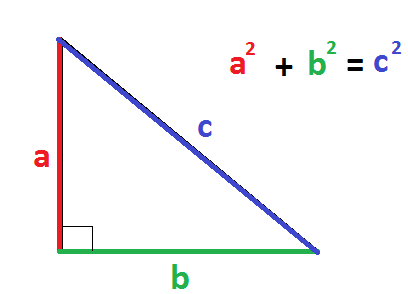# How do you find the length of the diagonal of a rectangle whose length is 6 meters and whose width is 4 meters in simple radical form?

May 29, 2018

The length of the diagonal is $2 \sqrt{13}$ meters or about $7.21$ meters in decimal form (rounded to nearest hundredth's place).

#### Explanation:

The diagonal of a rectangle plus two adjacent sides make a triangle. That "diagonal" is the same as the hypotenuse in a right triangle. Since we have the length and width and want the hypotenuse, we can use the Pythagorean Theorem shown below to solve it:Following this image, we know that
${\textcolor{red}{6}}^{2} + {\textcolor{\lim e g r e e n}{4}}^{2} = {\textcolor{b l u e}{c}}^{2}$

Simplify the left hand side:
$36 + 16 = {c}^{2}$

$52 = {c}^{2}$

Take the square root of both sides:
$\sqrt{52} = \sqrt{{c}^{2}}$

$c = \sqrt{52}$

#c = sqrt(4 * 13)

$c = \sqrt{4} \sqrt{13}$

$c = 2 \sqrt{13}$

The length of the diagonal is $2 \sqrt{13}$ meters or about $7.21$ meters in decimal form (rounded to nearest hundredth's place).

Hope this helps!